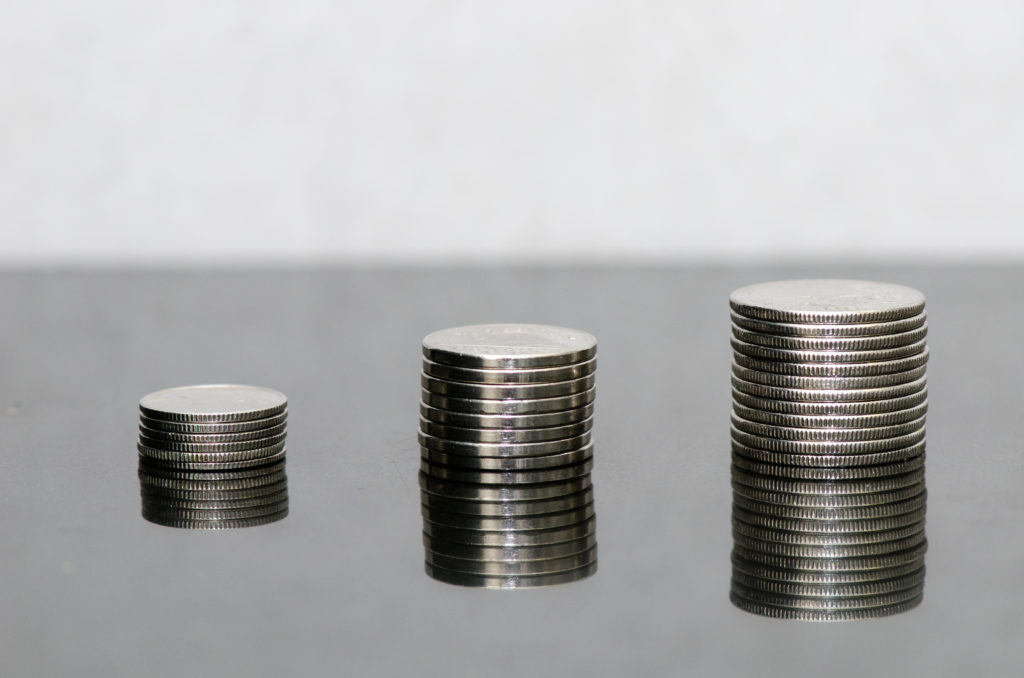## Solving Problems Involving Coins

### Learning Outcomes

• Solve coin word problems

Imagine taking a handful of coins from your pocket or purse and placing them on your desk. How would you determine the value of that pile of coins?

If you can form a step-by-step plan for finding the total value of the coins, it will help you as you begin solving coin word problems.

One way to bring some order to the mess of coins would be to separate the coins into stacks according to their value. Quarters would go with quarters, dimes with dimes, nickels with nickels, and so on. To get the total value of all the coins, you would add the total value of each pile.To determine the total value of a stack of nickels, multiply the number of nickels times the value of one nickel.

How would you determine the value of each pile? Think about the dime pile—how much is it worth? If you count the number of dimes, you’ll know how many you have—the number of dimes.

But this does not tell you the value of all the dimes. Say you counted $17$ dimes; how much are they worth? Each dime is worth $\text{\0.10}$ —that is the value of one dime. To find the total value of the pile of $17$ dimes, multiply $17$ by $\text{\0.10}$ to get $\text{\1.70}$. This is the total value of all $17$ dimes.

$\begin{array}{c}\hfill 17\cdot \text{\0.10}=\text{\1.70}\hfill \\ \hfill \text{number}\cdot \text{value}=\text{total value}\hfill \end{array}$

### Finding the Total Value for Coins of the Same Type

For coins of the same type, the total value can be found as follows:

$\text{number}\cdot \text{value}=\text{total value}$
where number is the number of coins, value is the value of each coin, and total value is the total value of all the coins.

You could continue this process for each type of coin, and then you would know the total value of each type of coin. To get the total value of all the coins, add the total value of each type of coin.

Let’s look at a specific case. Suppose there are $14$ quarters, $17$ dimes, $21$ nickels, and $39$ pennies. We’ll make a table to organize the information – the type of coin, the number of each, and the value.

Type $\text{Number}$ $\text{Value (\)}$ $\text{Total Value (\)}$
Quarters $14$ $0.25$ $3.50$
Dimes $17$ $0.10$ $1.70$
Nickels $21$ $0.05$ $1.05$
Pennies $39$ $0.01$ $0.39$
$6.64$

The total value of all coins is $\text{\6.64}$. Notice how the above table helped us organize the information.

Let’s review the problem-solving method for word problems.

### PROBLEM-SOLVING STRATEGY

Step 1. Read the problem. Make sure you understand all the words and ideas.

Step 2. Identify what you are looking for.

Step 3. Name what you are looking for.

Step 4. Translate into an equation. Restate the problem in one sentence. Then translate into an equation.

Step 5. Solve the equation using good algebra techniques.

Step 6. Check.

Let’s see how this method is used to solve a coin word problem.

### Example

Adalberto has $\text{\2.25}$ in dimes and nickels in his pocket. He has nine more nickels than dimes. How many of each type of coin does he have?

Solution:
Step 1. Read the problem. Make sure you understand all the words and ideas.

• Determine the types of coins involved.

Think about the strategy we used to find the value of the handful of coins. The first thing you need is to notice what types of coins are involved. Adalberto has dimes and nickels.

• Create a table to organize the information.
• Label the columns “type”, “number”, “value”, “total value”.
• List the types of coins.
• Write in the value of each type of coin.
• Write in the total value of all the coins.

### Example

Maria has $\text{\2.43}$ in quarters and pennies in her wallet. She has twice as many pennies as quarters. How many coins of each type does she have?

In the next example, we’ll show only the completed table—make sure you understand how to fill it in step by step.

### Example

Danny has $\text{\2.14}$ worth of pennies and nickels in his piggy bank. The number of nickels is two more than ten times the number of pennies. How many nickels and how many pennies does Danny have?

In the following video, we show another example of how to solve a word problem that involves finding an amount of coins.

## Contribute!

Did you have an idea for improving this content? We’d love your input.